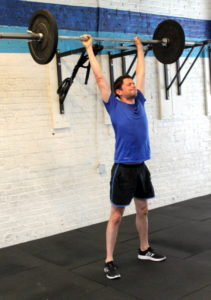## Friday, July 13, 2018

### Friday, July 13, 2018as many rounds as possible in seven minutes:
15x unbroken* wall @ 20/14-lbs., 10/9-ft. target
7x burpee
*Breaking a set of wall ball creates a 5x wall ball penalty, to be completed immediately. There will be a maximum of three penalties per round.

### 1 Comment

1.–Scores–
Borja G.: 6 rounds
Hari P.: 5 rounds + 1x burpee
Kate H.: 4 rounds + 15x wall ball (10-lbs., 10-ft.)
John L.: 5 rounds (14-lbs.)
Collin S.: 5 rounds + 10x wall ball)
Rami B.: 4 rounds + 6x burpee (14-lbs.)
Chris C.: 6 rounds + 5x wall ball
Chase H.: 6 rounds + 6x wall ball
David C.: 4 rounds + 15x wall ball (14-lbs.)
Daniel D.: 5 rounds + 11x wall ball (14-lbs.)
Adrian P.: 4 rounds + 10x wall ball (14-lbs.)
Amy S.: 6 rounds
Tara S.: 5 rounds + 6x burpee
Lauren M.: 5 rounds + 7x wall ball (10-lbs., 8-ft.; sub: wall ball)
Beth K.: 4 rounds + 1x burpee (10-lbs., 8/9-ft.)
Thomas R.: 4 rounds + 4x burpee
Cassie S.: 5 rounds + 15x wall ball
Dave R.: 7 rounds
Matthew W.: 7 rounds
Greg K.: 4 rounds + 6x wall ball
Elise C.: 4 rounds + 5x burpee (sub: box jump, 24″)
JP S.: 6 rounds + 5x pushup (sub: 20x air squat + 10x pushup)
Diana G.: 4 rounds + 5x wall ball (10-lbs., 8-ft.; sub: wall ball)
Eduardo S.: 5 rounds (14-lbs.)
Hirofumi J.: 4 rounds + 9x wall ball (10-lbs., 8-ft.; sub: wall ball)
Tiffany M.: 5 rounds, 1 penalty (14-lbs., 8-ft.)
Buffy M.: 4 rounds + 10x wall ball (10-lbs.; sub: prom)
Kerstin C.: 5 rounds (10-lbs., 8-ft.; sub: 2/3x wall ball, 1/2x burpee)
Jen M.: 6 rounds + 4x wall ball (10-lbs., 8-ft.; sub: 2/3x wall ball, 6/7x step-up, 18″)
Ralph B.: 5 rounds + 4x burpee
Joya TD.: 5 rounds + 4x wall ball (10-lbs.)
Lisa C.: 5 rounds + 10x wall ball (10-lbs.)
Rita S.: 3 rounds + 2x burpee (10-lbs.; sub: 5/7x burpee)
Yafet A.: 4 rounds (14-lbs.)
Mark S.: 5 rounds (14-lbs.)
RJ A.: 4 rounds, 3 penalty (14-lbs.)
Andrew B.: 5 rounds + 2x wall ball (14-lbs.)
Alex M.: 5 rounds + 13x wall ball
Elena P.: 4 rounds + 3x wall ball (10-lbs., 8-ft., sub: 2/3 wall ball)
Jamie Ac.: 4 rounds + 1x burpee (10-lbs., 8-ft.)
Julian M.: 5 rounds + 4x wall ball, 2 penalty
Charlie K.: 4 rounds + 8x wall ball
Sean Mc.: 4 rounds + 8 wall balls
Christian D.: 4 rounds + 15x wall ball (14-lbs.)
Josh M.: 5 rounds
Rachel B.: 5 rounds + 13x wall ball
Daniel C.: 4 rounds + 13x wall ball
Kit H.: 6 rounds + 3x wall ball
Jared F.: 4 rounds
Max M.: 5 rounds
Mark Co.: 5 rounds
Melody F.: 6 rounds
Marcus B.: 5 rounds + 5x wall ball